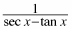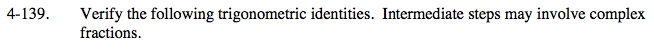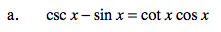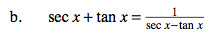### Home > PC > Chapter 4 > Lesson 4.3.2 > Problem4-139

4-139.
1. Verify the following trigonometric identities. Intermediate steps may involve complex fractions. Homework Help ✎

1. csc x − sin x = cot x cos x

2. sec x + tan x =1. Change all terms to sine and cosine.
2. Get a common denominator.1. Change all terms to sine and cosine.
2. Get a common denominator.
3. Use the Fundamental Pythagorean Identities.